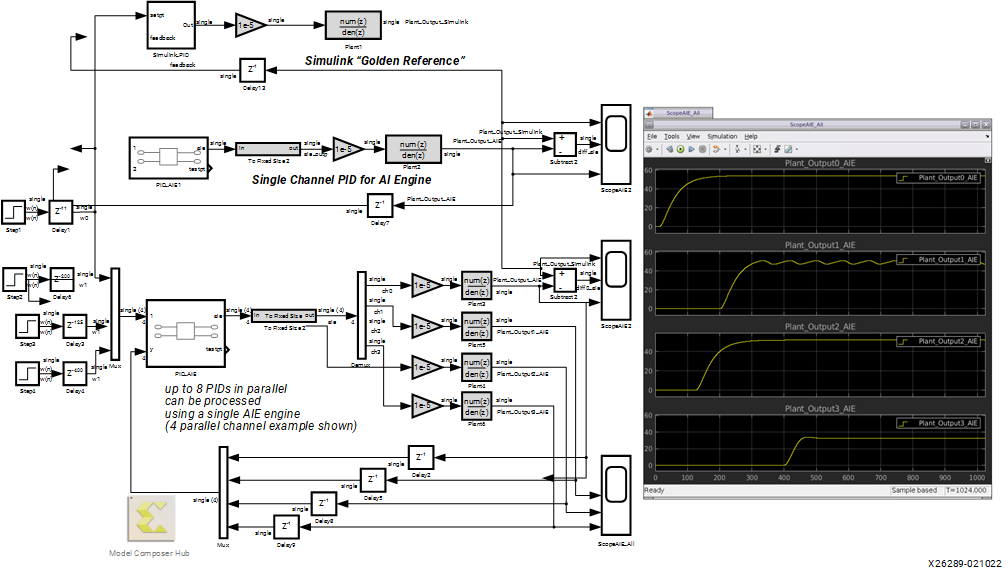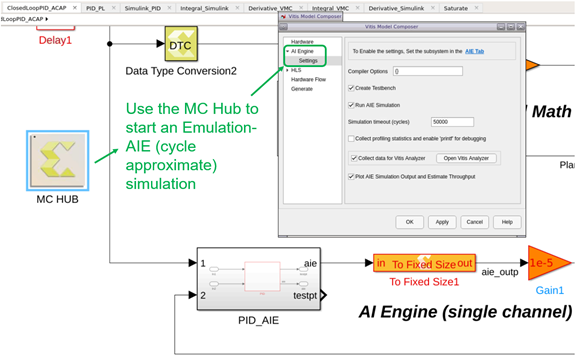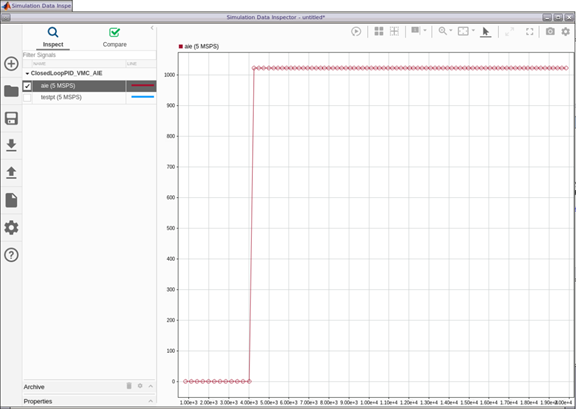# VMC AI Engine Design

## PID Controller Design with Model Composer for Versal ACAPs (XAPP1376)

Document ID
XAPP1376
Release Date
2022-03-09
Revision
1.0 English

AI Engines add another flexible dimension to numerical computations. In order to show the versatility of the Versal AI Engine, the PID was re-written to target an AI Engine. A single channel SPFP AI Engine based PID intrinsic source code is shown in the following code.

``````
// error = setpt - feedback
error = upd_elem(error, 0, readincr(setpt)); // MM 1/6 was inp_data, 0, readincr...
error = fpsub(error, scratch_pad); // save error data

// proportional code
acc = fpmul(error, *Gp_ptr); // acc now holds proportional path results

writeincr(testpt, ext_elem(error, 0)); // MM

// derivative code
inp_data = fpmul(error, *Gd_ptr); // X1(n)
scratch_pad = fpsub( inp_data, fpmul(derivative_delay1, *C_ptr) ); // X1(n)-CYd(n-1)
derivative_delay = inp_data;

// add proportional & derivative results

// integral code
inp_data = fpmul(error, *Gi_ptr); // X2(n)
integral_delay = inp_data;

// test for saturation for integral path (ie: prevent integral anti-windup)
else if (ext_elem(scratch_pad,0) < min_clip )

// add proportional, integral, derivative results

// test for saturation
if (ext_elem(acc,0) > max_clip)
acc = upd_elem(acc, 0, max_clip);
else if (ext_elem(acc,0) < min_clip )
acc = upd_elem(acc, 0, min_clip);

// write out results for servo lane 0
writeincr(outp, ext_elem(acc, 0));

}``````

A single channel PID implementation only utilizes one -eighth of the full AI Engine capacity. Alternately, the vector processor’s single instruction multiple data (SIMD) capability can be used to process between one and eight PIDs in parallel. The following figure is an example of both a single channel (reference PID.cc source code) and four channel (reference PID_rv2.cc source code) SPFP PIDs running concurrently on an AI Engine (reference source code: PID_rv2.cc).

Figure 1. Four Channel AI Engine PID Single Channel PID Compared to a Single Channel AI Engine PID and the Simulink Golden Reference (Reference Design: ClosedLoopPID_ACAP_rv2.slx)The four channel scope results (ScopeAIE_All) display the results for four different sets of Kp, Ki, and Kd coefficients. The C++ for the AI Engine was functionally debugged during development via Vitis Emulation-SW simulations executed by pushing the Simulink run button.

Figure 2. Functionally Simulating (Vitis Emulation-SW Simulation) an AI Engine Design in SimulinkFunctional debugging (Vitis Emulation-SW simulation) can be one to two orders of magnitude faster than running the same models using cycle approximate simulations (Vitis Emulation-AI Engine simulations). Therefore, a large part of development should use functional simulations in order to reduce development time and simplify debug of any new design. After functional verification of the PID controller completes, the Vitis Emulation-AI Engine (cycle approximate) simulator is used via the MC Hub token as demonstrated in the following figure.

Figure 3. Running the Bit Accurate and Cycle Approximate (Emulation-AI Engine) SimulationCycle approximate simulations allow improved throughput by changing the source code or applying compiler directives and debugging potential cycle accurate implementation issues. When the Model Composer Hub is used for cycle approximate simulation, the following automated steps are performed:

1. A test bench using the Simulink design is created, and adaptive dataflow graph (ADF) is generated.
2. The Emulation-AI Engine Vitis flow is run using the Vitis tools.
3. The Vitis analyzer opens for detailed analysis.
4. The Emulation-AI Engine simulation output is plotted and estimates the throughput.

Plotting the cycle approximate (Emulation-AI Engine simulation) output estimates for the single-channel AI Engine based PID design has a 5 MSPS throughput as shown in the following figure.

Figure 4. Emulation-AI Engine Throughput EstimatesThe four-channel AI Engine PID has a 4 MSPS throughput. The difference in sample rate performance between the single-channel and four-channel PID is the conditional statements necessary to iterate across four parallel channels. Line 105 in PID_rv2.cc has a constant `num_pids` which defines the PIDs for loop lengths. The existing value is four, but the maximum value is eight. For the sake of simplicity, and to keep the Simulink ADF sheet from being too cluttered for explanation purposes, an arbitrary four channels was chosen.